# Problem: Part A.)Determine the direction of the force on the charge due to the magnetic field. (Figure 1). a)  points into the page.b)  points out of the page.c)  points neither into nor out of the page and .d) .Part B.)Determine the direction of the force on the charge due to the magnetic field. (Figure 2)a)  points out of the page.b)  points into the page.c) points neither into nor out of the page and .d) .Part C.)Determine the direction of the force on the charge due to the magnetic field. Note that the charge is negative. (Figure 3)a)  points out of the page.b)  points into the page.c)  points neither into nor out of the page and .d) .

###### FREE Expert Solution

Part A

Force on a charged particle:

$\overline{){\mathbf{F}}{\mathbf{=}}{\mathbf{q}}{\mathbf{v}}{\mathbf{B}}{\mathbf{s}}{\mathbf{i}}{\mathbf{n}}{\mathbf{\theta }}}$

θ = 180°

83% (115 ratings)###### Problem Details

Part A.)

Determine the direction of the force on the charge due to the magnetic field. (Figure 1)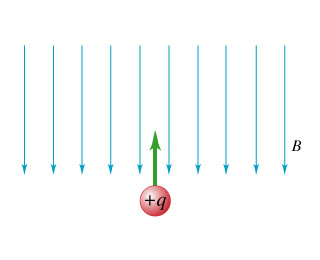a)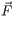points into the page.

b)points out of the page.

c)points neither into nor out of the page and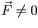.

d)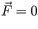.

Part B.)

Determine the direction of the force on the charge due to the magnetic field. (Figure 2)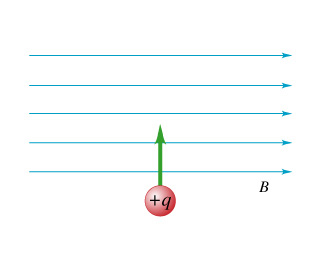a)points out of the page.

b)points into the page.

c)points neither into nor out of the page and.

d).

Part C.)

Determine the direction of the force on the charge due to the magnetic field. Note that the charge is negative. (Figure 3)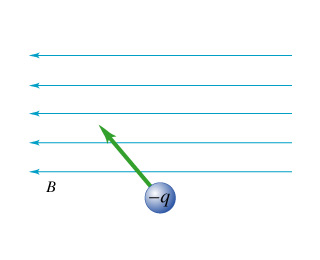a)points out of the page.

b)points into the page.

c)points neither into nor out of the page and.

d).